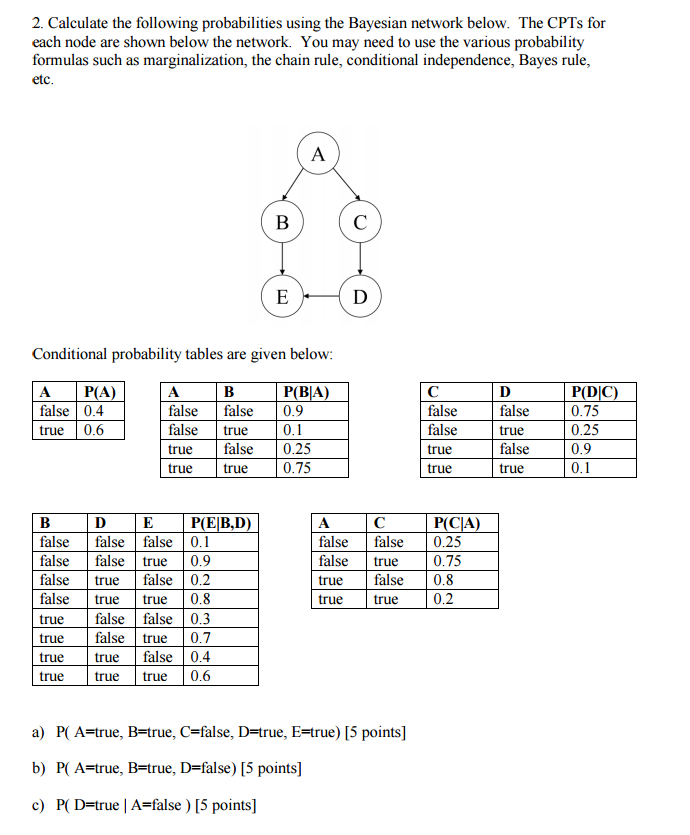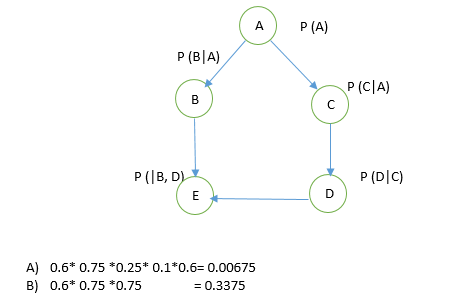# Question & Answer: Calculate the following probabilities using the Bayesian network below. The CPTs for each node are shown…..

Calculate the following probabilities using the Bayesian network below. The CPTs for each node are shown below the network. You may need to use the various probability formulas such as marginalization, the chain rule, conditional independence, Bayes rule, etc. Conditional probability tables are given below: a) P(A=true, B=true, C=false, D=true, E=true) b) P(A=true, B=true, D=false) c) P(D=true | A=false)